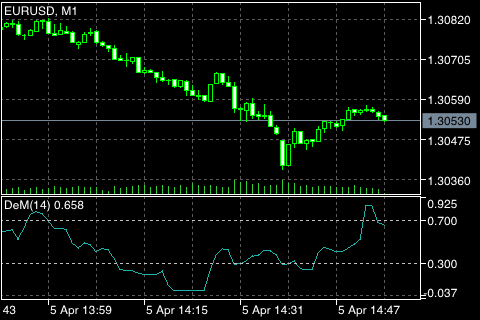# 迪马克 (DeMarker)## 计算

DeMax(i) 的计算。如果 HIGH (i) > HIGH (i - 1)，那么:

DeMax (i) = HIGH (i) - HIGH (i - 1)

DeMax (i) = 0

DeMin(i) 的计算。如果 LOW (i) < LOW (i - 1), 则:

DeMin (i) = LOW (i - 1) - LOW (i)

DeMin (i) = 0

DMark (i) = SMA (DeMax, N) / (SMA (DeMax, N) + SMA (DeMin, N))

HIGH (i) ― 当前柱的最高价格;
LOW (i) ― 当前柱的最低价格;
HIGH (i - 1) ― 上一时段的最高价格;
LOW (i - 1) ― 上一时段的最低价格;
SMA ― 简单移动平均线;
N ― 计算中使用到的周期。